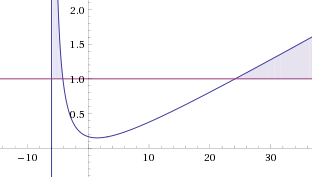# Quadratic With Inequality Insane Level!

Algebra Level 5The values of $k$ for which the inequality $\dfrac{x^2+k^2}{k \cdot (x+6)} \geq 1$ is satisfied by all $x \in (-1,1)$ are $[a, \infty)$.

Find the value of $a$ (round off up to 3 decimal places).

×